24, Ground Floor, Rameswaram Road, T.Nagar, Chennai -600 017. Phone: 044 2432 246

#### Best Customer Support

Call:044 22501105 (Guindy),
044 24322469 (T. Nagar)

#### Reasonable price## Best Sellers

Shop nowShop now

## Complex Analysis - B.Sc - S.G. Venkatachalapathy

• Complex Numbers
• The Geometric Representation of Complex Numbers
• Limits and Continuity
• Analytic Functions
• Conformal Mapping
• Infinite Series, Power Series
• Complex Integration
• Development of Analytic Functions as Power Series
• Singularities
• Calculus of Residues
Book Author S.G. Venkatachalapathy Margham Publications English B.Sc. Mathematics, Madras and other South Indian Universities.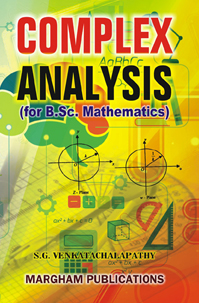₹200.00

## Complex Analysis - M.Sc - S.G. Venkatachalapathy

• Complex Numbers
• The Geometric Representation of Complex Numbers
• The Exponential and Trigonometric Functions
• Analytic Functions as Mappings
• Complex Integration
Book Author S.G. Venkatachalapathy Margham Publications English M.Sc. Mathematics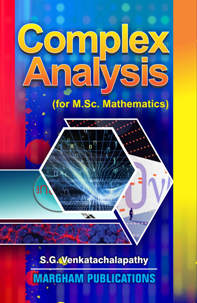₹180.00

## Differential Equations, Fourier & Laplace Transforms, Probability - Dr. P.R. Vittal

• (A) First Order and First Degree Equations of Bernoulli (B) Differential Equations of First Order and Higher Degree
• Second Order Differential Equations with Constant Coefficients
• Linear Homogeneous Equations and Variation of Parameters
• Total Differential Equations
• Partial Differential Equations
• Fourier Series
• Laplace Transform
• Fourier Transform
• Solutions of Differential Equations by Power Series Method
Book Author P.R. Vittal Margham Publications English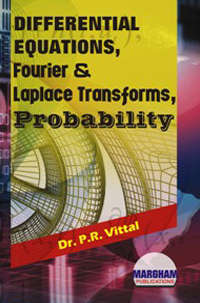₹200.00

## Differential Equations & Laplace Transforms III Semester - P.R. Vittal

• (A) First Order and First Degree Equations of Bernoulli
• (B) Differential Equations of First Order and Higher Degree
• Second Order Differential Equations with constant Coefficients
• Linear Homogeneous Equation and Variation of Parameters
• Total Differential Equations
• Partial Differential Equations
• Laplace Transform
Book Author P.R. Vittal Margham Publications English B.Sc., Mathematics Major - Madras University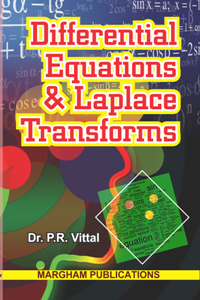₹150.00

## Differential Geometry - S.G. Venkatachalapathy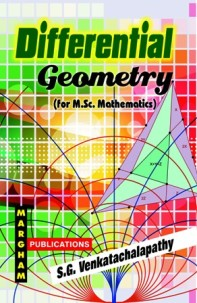₹140.00

Page 5 of 11